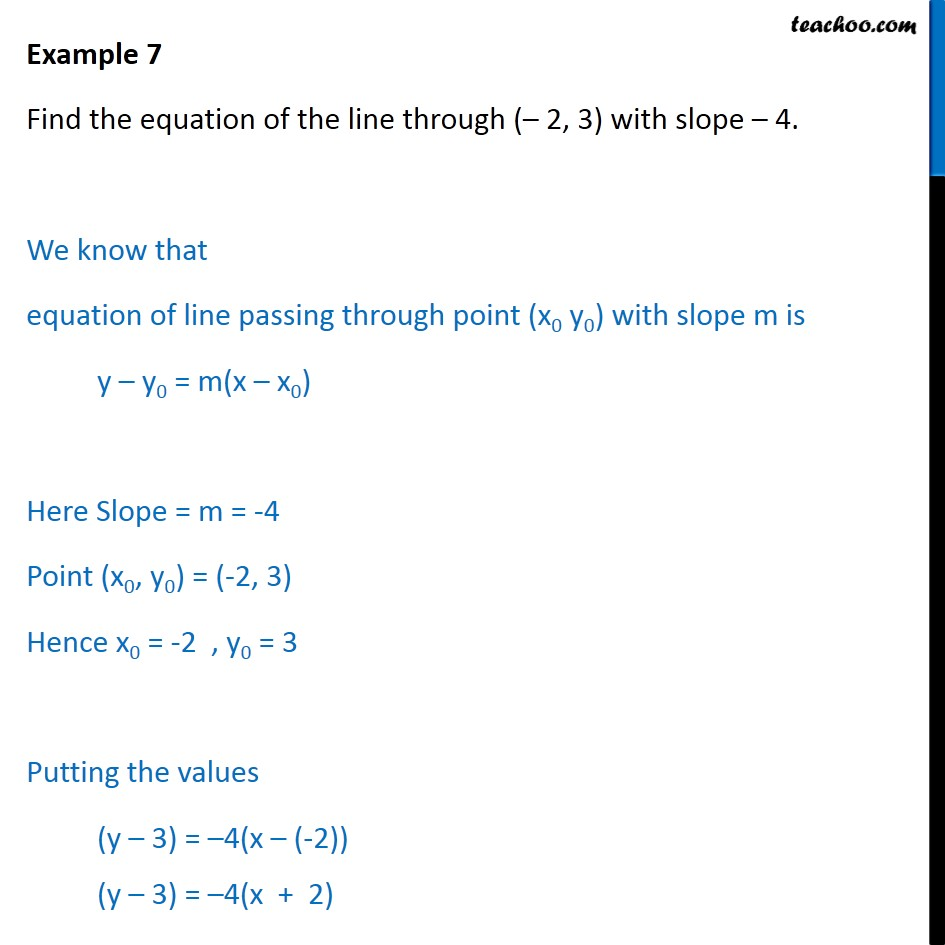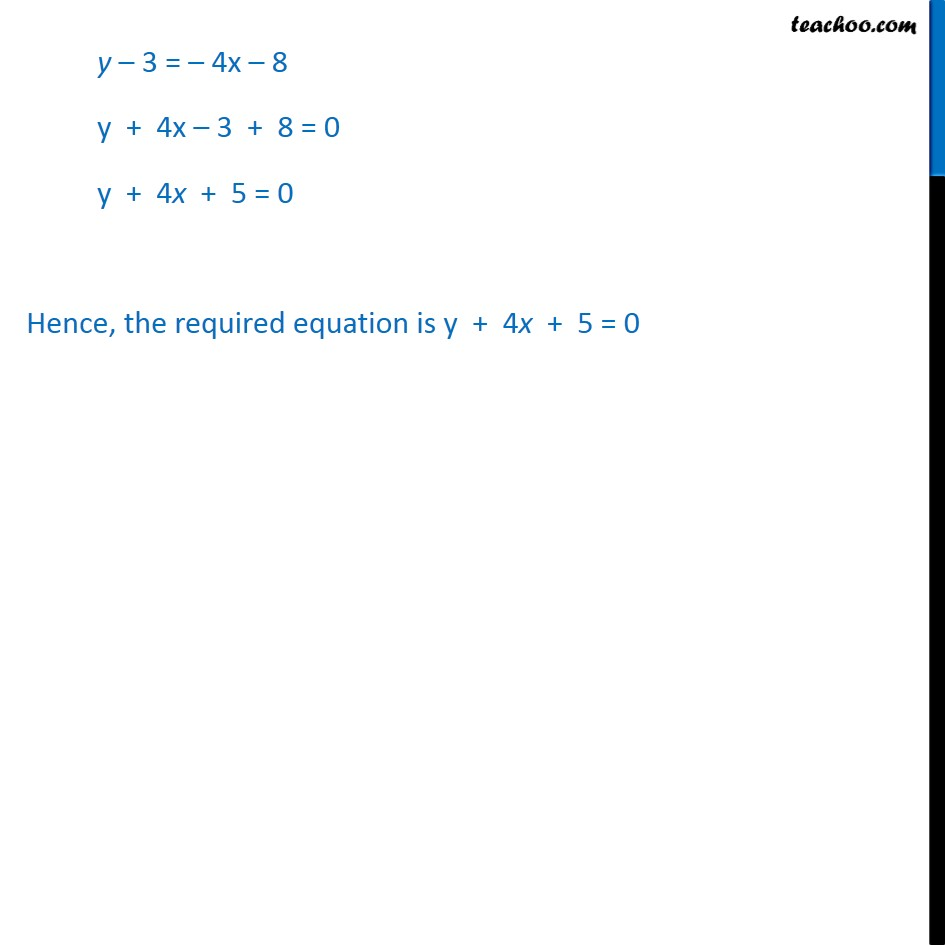Examples

Chapter 9 Class 11 Straight Lines
Serial order wiseLearn in your speed, with individual attention - Teachoo Maths 1-on-1 Class

### Transcript

Example 5 Find the equation of the line through (– 2, 3) with slope – 4. We know that equation of line passing through point (x0 y0) with slope m is y – y0 = m(x – x0) Here Slope = m = -4 Point (x0, y0) = (-2, 3) Hence x0 = -2 , y0 = 3 Putting the values (y – 3) = –4(x – (-2)) (y – 3) = –4(x + 2) y – 3 = – 4x – 8 y + 4x – 3 + 8 = 0 y + 4x + 5 = 0 Hence, the required equation is y + 4x + 5 = 0